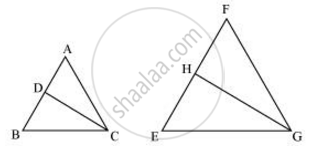# CD and GH are respectively the bisectors of ∠ACB and ∠EGF such that D and H lie on sides AB and FE of ΔABC and ΔEFG respectively. If ΔABC ~ ΔFEG, Show that - Mathematics

CD and GH are respectively the bisectors of ∠ACB and ∠EGF such that D and H lie on sides AB and FE of ΔABC and ΔEFG respectively. If ΔABC ~ ΔFEG, Show that

(i) (CD)/(GH) = (AC)/(FG)

(ii) ΔDCB ~ ΔHGE

(iii) ΔDCA ~ ΔHGF

#### SolutionIt is given that ΔABC ∼ ΔFEG.

∴ ∠A = ∠F, ∠B = ∠E, and ∠ACB = ∠FGE

∠ACB = ∠FGE

∴ ∠ACD = ∠FGH (Angle bisector)

And, ∠DCB = ∠HGE (Angle bisector)

In ΔACD and ΔFGH,

∠A = ∠F (Proved above)

∠ACD = ∠FGH (Proved above)

∴ ΔACD ∼ ΔFGH (By AA similarity criterion)

=>(CD)/(GH) = (AC)/(FG)

In ΔDCB and ΔHGE,

∠DCB = ∠HGE (Proved above)

∠B = ∠E (Proved above)

∴ ΔDCB ∼ ΔHGE (By AA similarity criterion)

In ΔDCA and ΔHGF,

∠ACD = ∠FGH (Proved above)

∠A = ∠F (Proved above)

∴ ΔDCA ∼ ΔHGF (By AA similarity criterion)

Concept: Criteria for Similarity of Triangles
Is there an error in this question or solution?

#### APPEARS IN

NCERT Class 10 Maths
Chapter 6 Triangles
Exercise 6.3 | Q 10 | Page 140